# P vs. NP — What is the Difference Between Solving a Problem and Recognizing its Solution?

## Diving into the most notorious open question in computer science and its far-reaching philosophical consequences.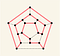A dodecahedron as a two-dimensional planar graph. One possible Hamiltonian cycle is shown in red.

Given a Graph, determine whether it contains an Hamiltonian cycle.

“The asymptotic viewpoint is inherent to computational complexity theory, and reveals structure which would be obscured by finite, precise analysis.”

— Avi Wigderson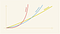An algorithm’s Time Complexity is described as an asymptotical function that is dependent on the algorithm’s input size. A main distinction is made between a Factorial, Exponential, and Polynomial complexity functions.

Algorithms that have a Polynomial Time Complexity are referred to as “efficient”.

A Decision Problem is in NP if its solutions can be efficiently verified.

P is the set of all decision problems that are efficiently solvable. P is a subset of NP.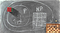P is the set of all decision problems that are efficiently solvable and is a subset of NP. Basic Arithmetic is solvable in Polynomial-time, thus belongs to P. Soduko’s decision problem is in NP, whereas it is not clear whether it is in P. Chess decision problem is outside of NP since there is no efficient algorithm that can even check whether a given chess board is valid. Rubik’s Cube decision problem is in NP because it is trivial to tell whether a given cube is a solution. In contrast, there is no knowledge of an efficient algorithm that can solve this problem. Source: hackerdashery.

Do P and NP are actually the same? If Yes, it means that all the problems in NP can be efficiently solved even though we still haven’t found the mysterious algorithms that achieve that. Otherwise, there are problems in NP that are impossible to solve efficiently, and any attempt to do so would mean a complete waste of our time and efforts.

Given two positive integers n and k, decide whether n has a prime factor smaller than k.

— The Prime Factorization Decision Problem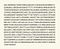RSA-2048 has 617 decimal digits (2,048 bits). It is the largest of the RSA numbers and carried the largest cash prize for its factorization, \$200,000. The RSA-2048 may not be factorizable for many years to come unless considerable advances are made in integer factorization or computational power in the near future.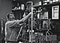Christian Boehmer Anfinsen in his lab. He was one of the 1972 winners of the Nobel Prize in Chemistry for his efforts to study the folding of a small, hardy 100-amino-acid-long protein called ribonuclease A. The Protein Folding Problem is an NP problem.

If T is a theorem and p is its proof, then p is a solution for the decision problem: “Is T correct?”

P equals NP may imply that proving a mathematical theorem is not fundamentally harder than verifying the correctness of its proof.

“P equals NP would transform mathematics by allowing a computer to find a formal proof of any theorem which has a proof of a reasonable length, since formal proofs can easily be recognized in polynomial time.”

— Stephen Cook

“We admire Wiles’ proof of Fermat’s Last Theorem, the scientific theories of Newton, Einstein, Darwin, Watson and Crick, the design of the Golden Gate bridge and the Pyramids, and sometimes even Hercule Poirot’s and Miss Marple’s analysis of a murder, precisely because they seem to require a leap which cannot be made by everyone, let alone by a simple mechanical device”

— Avi Wigderson

“If P = NP, any human or computer would have the sort of reasoning power traditionally ascribed to deities, and this seems hard to accept.”

— Avi Wigderson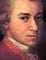Mozart, c. 1781, detail from portrait by Johann Nepomuk della Croce

“If P=NP, then the world would be a profoundly different place than we usually assume it to be. Everyone who could appreciate a symphony would be Mozart.”

— Scott Aaronson

Written by

Written by

## Eliran Natan

#### Creating Reactive Apps that shape Human lives, focusing on IoT and Progressive Web Experiences. I share code and experiences on theHardcoded.blog